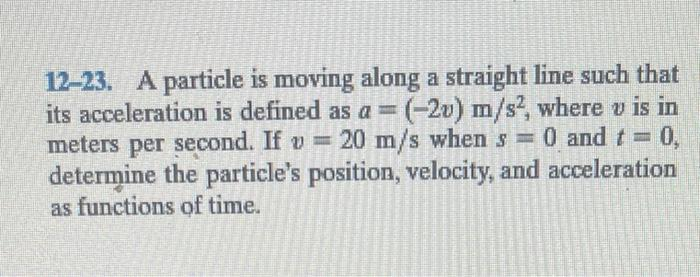Home / Expert Answers / Mechanical Engineering / 12-23-a-particle-is-moving-along-a-straight-line-such-that-its-acceleration-is-defined-as-a-2-pa571

# (Solved): 12-23. A particle is moving along a straight line such that its acceleration is defined as $$a=(-2 ...12-23. A particle is moving along a straight line such that its acceleration is defined as \( a=(-2 v) \mathrm{m} / \mathrm{s}^{2}$$, where $$v$$ is in meters per second. If $$v=20 \mathrm{~m} / \mathrm{s}$$ when $$s=0$$ and $$t=0$$, determine the particle's position, velocity, and acceleration as functions of time.

We have an Answer from Expert